Text geoms are useful for labeling plots. They can be used by themselves as scatterplots or in combination with other geoms, for example, for labeling points or for annotating the height of bars. geom_text() adds only text to the plot. geom_label() draws a rectangle behind the text, making it easier to read.

geom_label(
mapping = NULL,
data = NULL,
stat = "identity",
position = "identity",
...,
parse = FALSE,
nudge_x = 0,
nudge_y = 0,
label.r = unit(0.15, "lines"),
label.size = 0.25,
na.rm = FALSE,
show.legend = NA,
inherit.aes = TRUE
)

geom_text(
mapping = NULL,
data = NULL,
stat = "identity",
position = "identity",
...,
parse = FALSE,
nudge_x = 0,
nudge_y = 0,
check_overlap = FALSE,
na.rm = FALSE,
show.legend = NA,
inherit.aes = TRUE
)

## Arguments

mapping Set of aesthetic mappings created by aes() or aes_(). If specified and inherit.aes = TRUE (the default), it is combined with the default mapping at the top level of the plot. You must supply mapping if there is no plot mapping. The data to be displayed in this layer. There are three options: If NULL, the default, the data is inherited from the plot data as specified in the call to ggplot(). A data.frame, or other object, will override the plot data. All objects will be fortified to produce a data frame. See fortify() for which variables will be created. A function will be called with a single argument, the plot data. The return value must be a data.frame, and will be used as the layer data. A function can be created from a formula (e.g. ~ head(.x, 10)). The statistical transformation to use on the data for this layer, as a string. Position adjustment, either as a string, or the result of a call to a position adjustment function. Cannot be jointy specified with nudge_x or nudge_y. Other arguments passed on to layer(). These are often aesthetics, used to set an aesthetic to a fixed value, like colour = "red" or size = 3. They may also be parameters to the paired geom/stat. If TRUE, the labels will be parsed into expressions and displayed as described in ?plotmath. Horizontal and vertical adjustment to nudge labels by. Useful for offsetting text from points, particularly on discrete scales. Cannot be jointly specified with position. Amount of padding around label. Defaults to 0.25 lines. Radius of rounded corners. Defaults to 0.15 lines. Size of label border, in mm. If FALSE, the default, missing values are removed with a warning. If TRUE, missing values are silently removed. logical. Should this layer be included in the legends? NA, the default, includes if any aesthetics are mapped. FALSE never includes, and TRUE always includes. It can also be a named logical vector to finely select the aesthetics to display. If FALSE, overrides the default aesthetics, rather than combining with them. This is most useful for helper functions that define both data and aesthetics and shouldn't inherit behaviour from the default plot specification, e.g. borders(). If TRUE, text that overlaps previous text in the same layer will not be plotted. check_overlap happens at draw time and in the order of the data. Therefore data should be arranged by the label column before calling geom_text(). Note that this argument is not supported by geom_label().

## Details

Note that when you resize a plot, text labels stay the same size, even though the size of the plot area changes. This happens because the "width" and "height" of a text element are 0. Obviously, text labels do have height and width, but they are physical units, not data units. For the same reason, stacking and dodging text will not work by default, and axis limits are not automatically expanded to include all text.

geom_text() and geom_label() add labels for each row in the data, even if coordinates x, y are set to single values in the call to geom_label() or geom_text(). To add labels at specified points use annotate() with annotate(geom = "text", ...) or annotate(geom = "label", ...).

To automatically position non-overlapping text labels see the ggrepel package.

## Aesthetics

geom_text() understands the following aesthetics (required aesthetics are in bold):

• x

• y

• label

• alpha

• angle

• colour

• family

• fontface

• group

• hjust

• lineheight

• size

• vjust

Learn more about setting these aesthetics in vignette("ggplot2-specs").

## geom_label()

Currently geom_label() does not support the check_overlap argument or the angle aesthetic. Also, it is considerably slower than geom_text(). The fill aesthetic controls the background colour of the label.

## Alignment

You can modify text alignment with the vjust and hjust aesthetics. These can either be a number between 0 (right/bottom) and 1 (top/left) or a character ("left", "middle", "right", "bottom", "center", "top"). There are two special alignments: "inward" and "outward". Inward always aligns text towards the center, and outward aligns it away from the center.

## Examples

p <- ggplot(mtcars, aes(wt, mpg, label = rownames(mtcars)))

p + geom_text()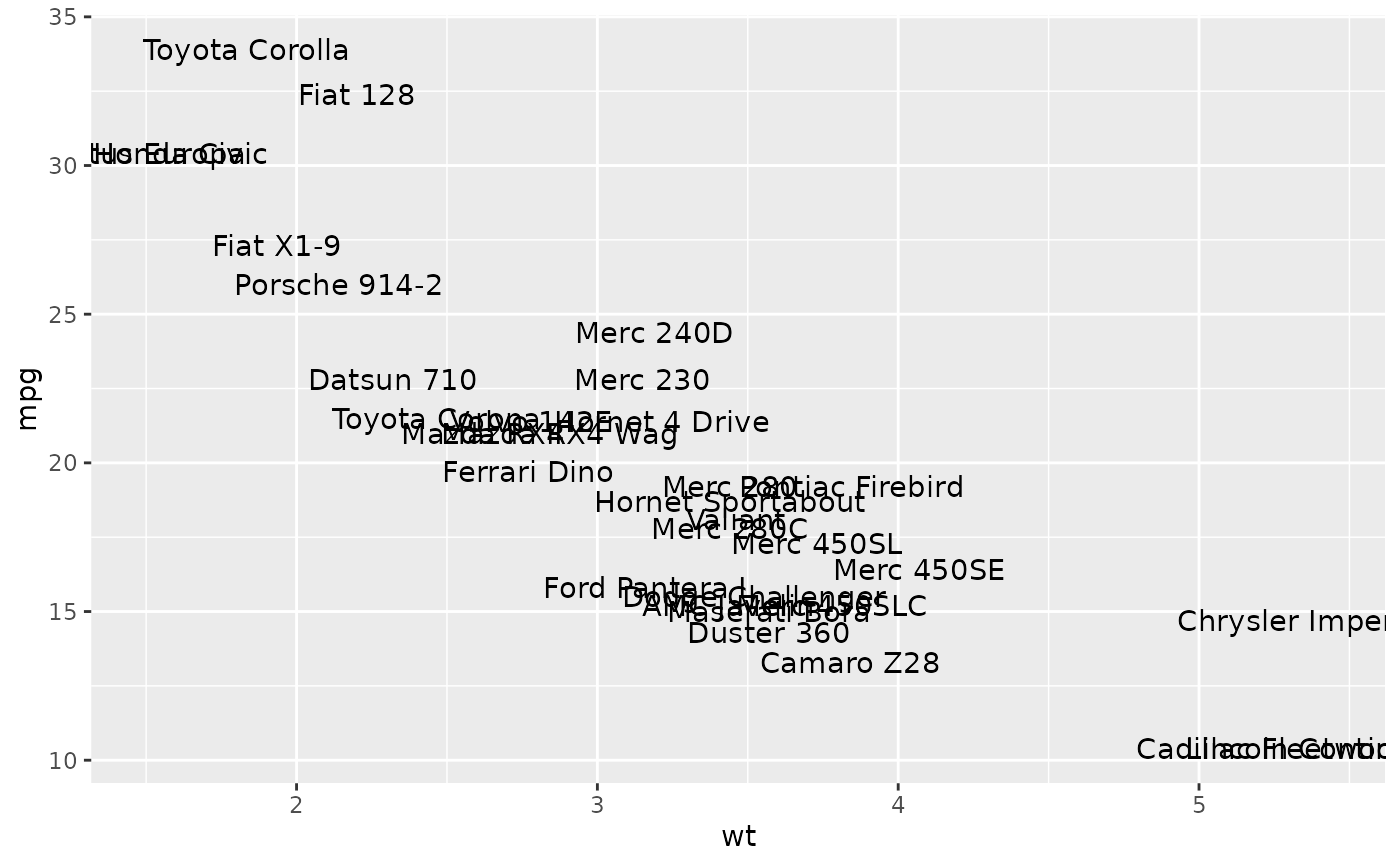# Avoid overlaps
p + geom_text(check_overlap = TRUE)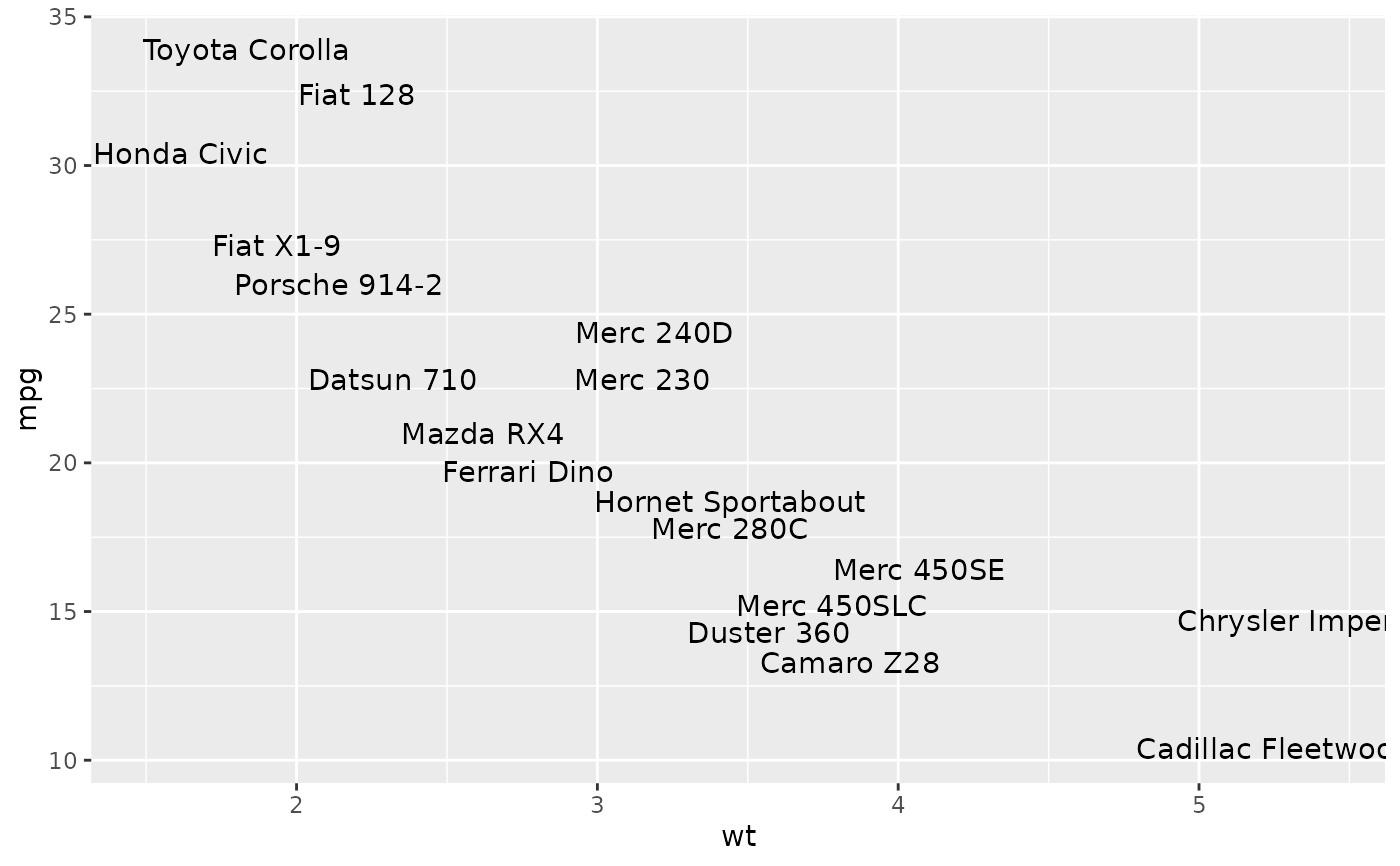# Labels with background
p + geom_label()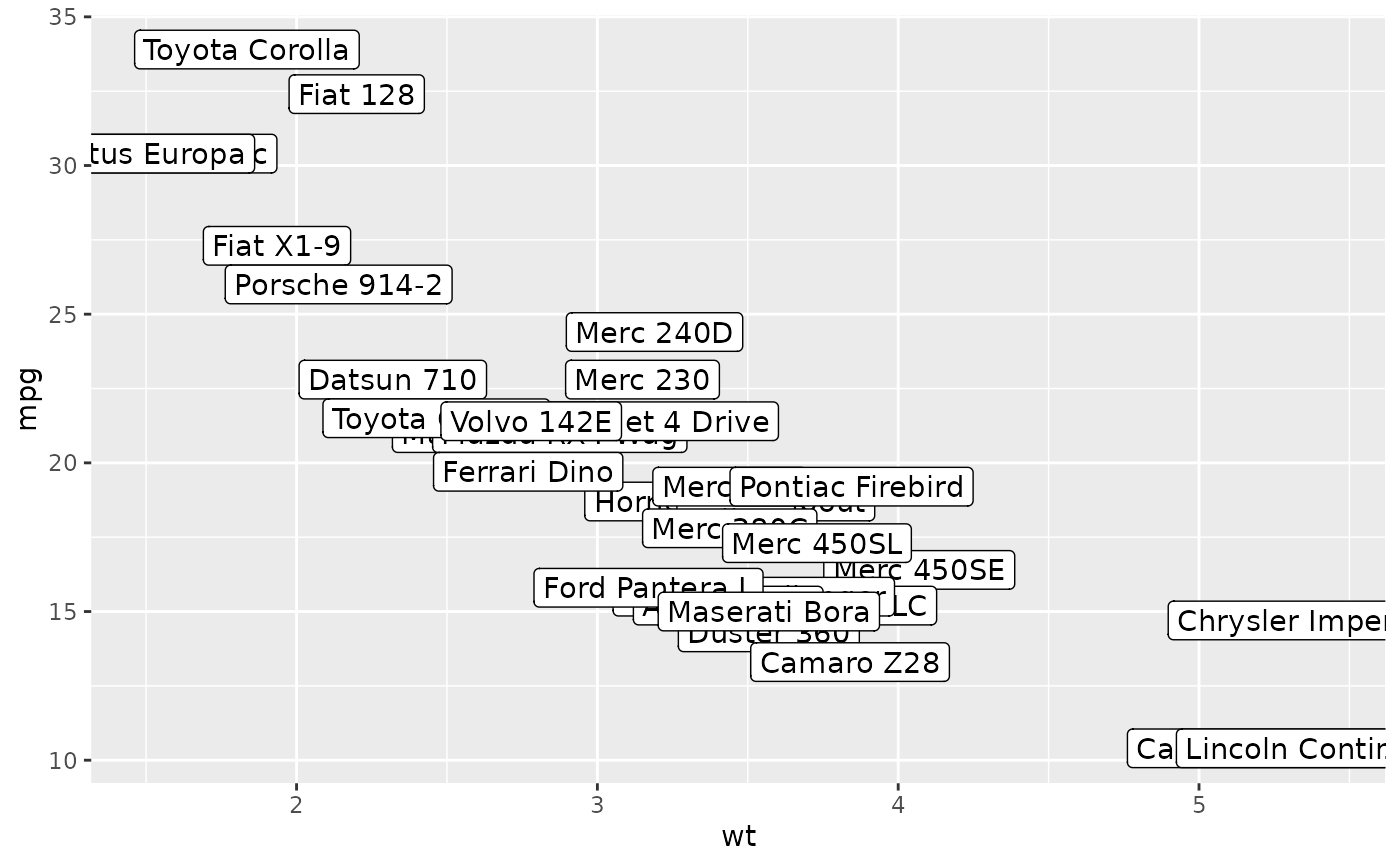# Change size of the label
p + geom_text(size = 10)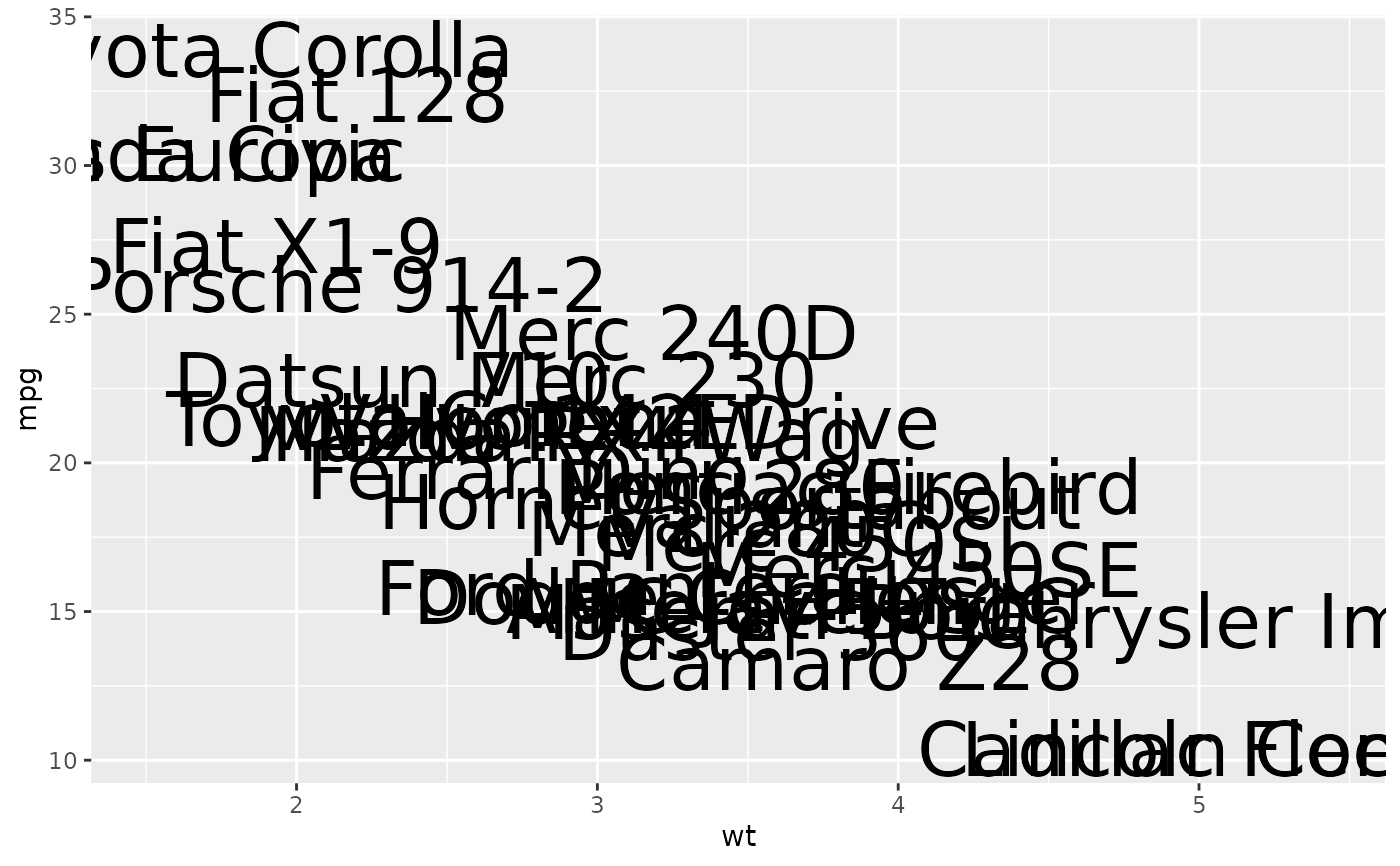# Set aesthetics to fixed value
p +
geom_point() +
geom_text(hjust = 0, nudge_x = 0.05)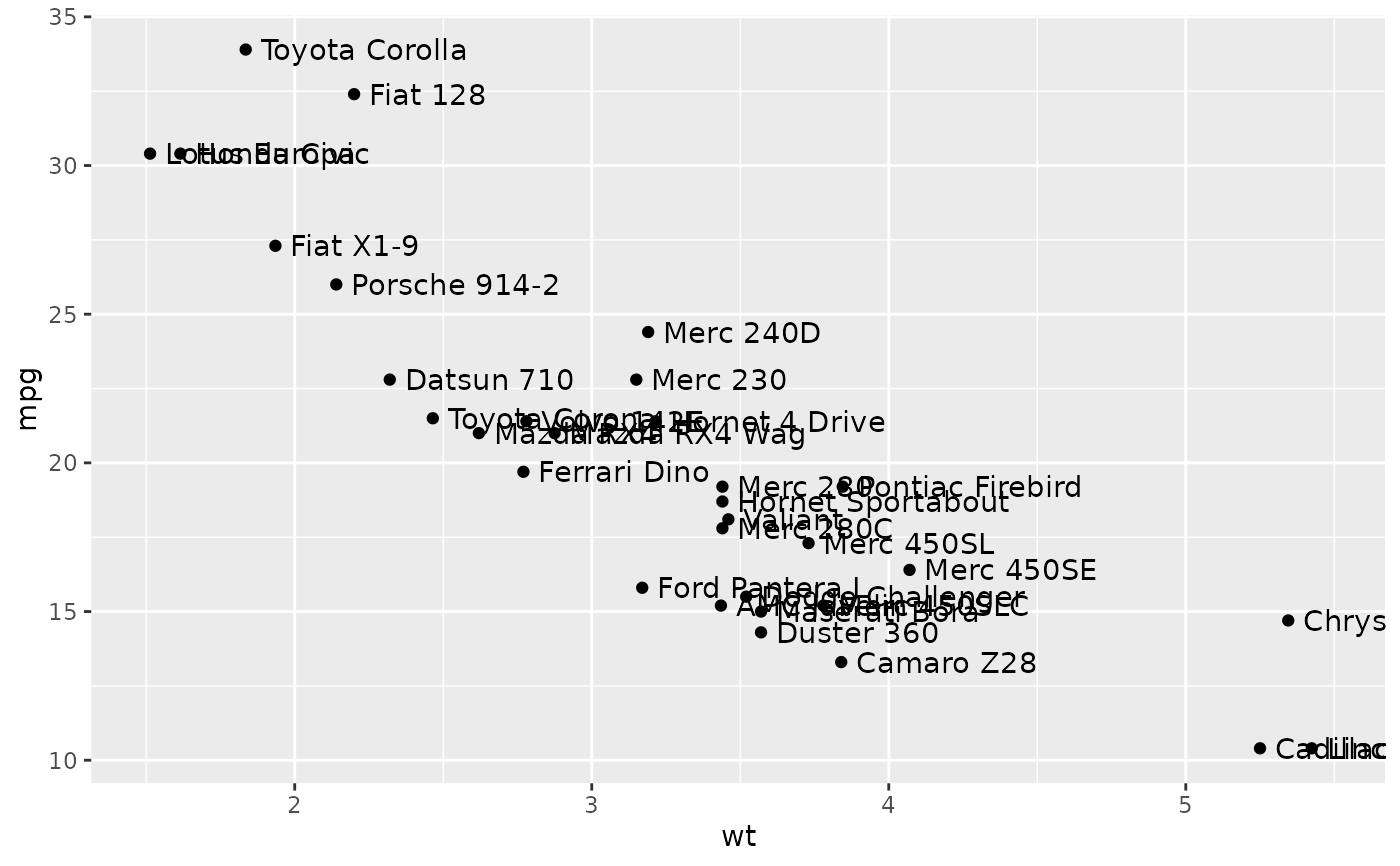p +
geom_point() +
geom_text(vjust = 0, nudge_y = 0.5)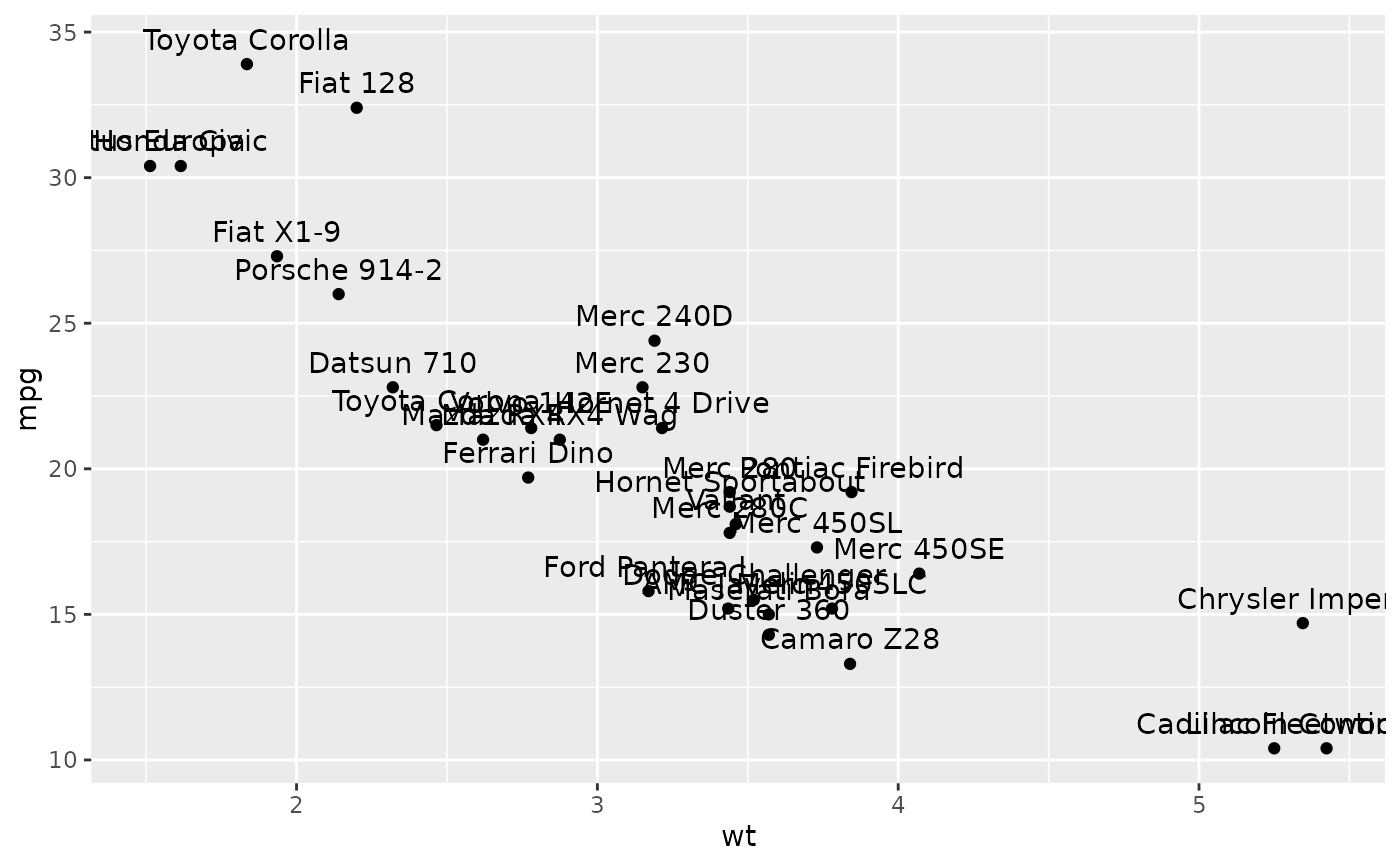p +
geom_point() +
geom_text(angle = 45)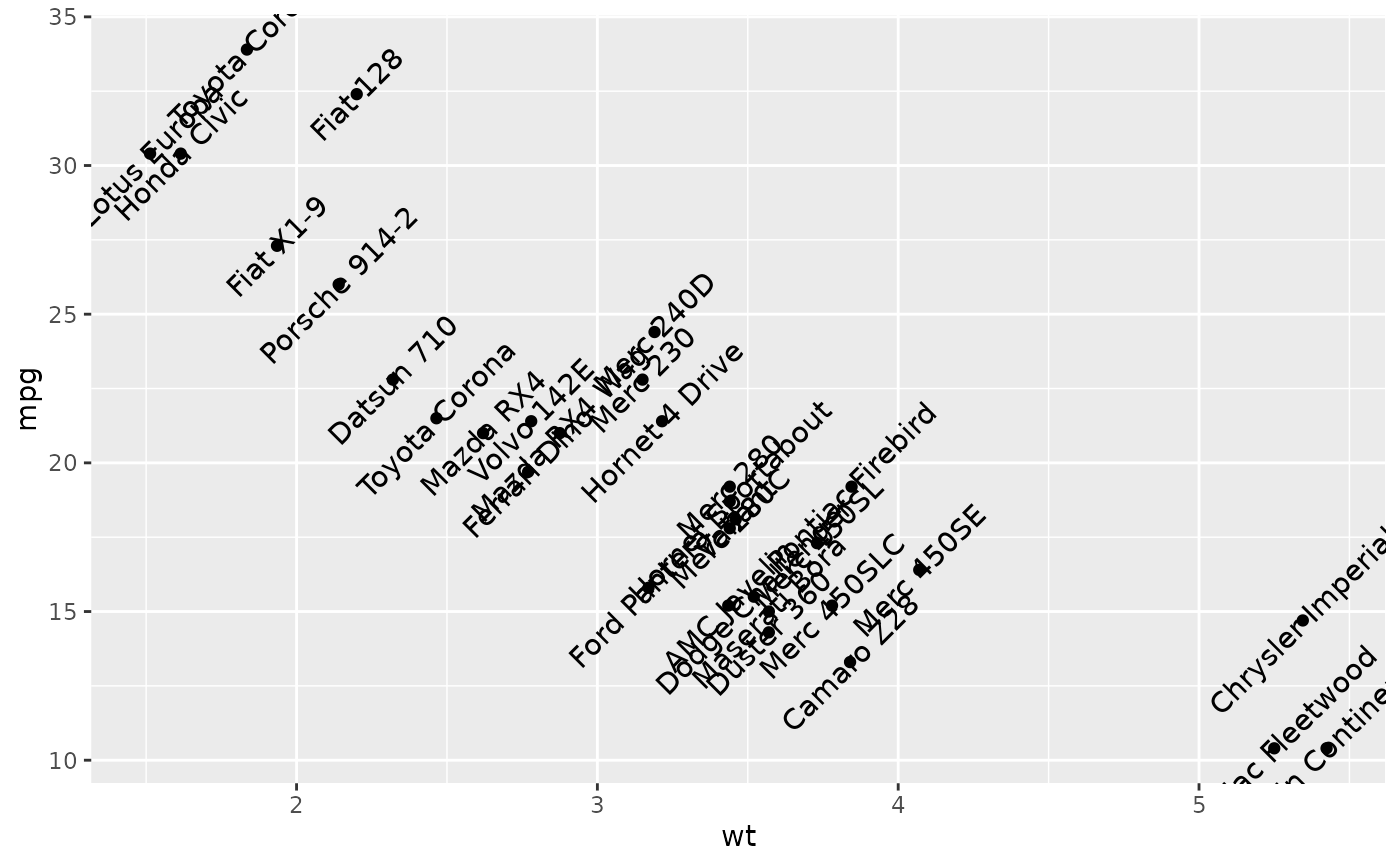if (FALSE) {
# Doesn't work on all systems
p +
geom_text(family = "Times New Roman")
}

p + geom_text(aes(colour = factor(cyl)))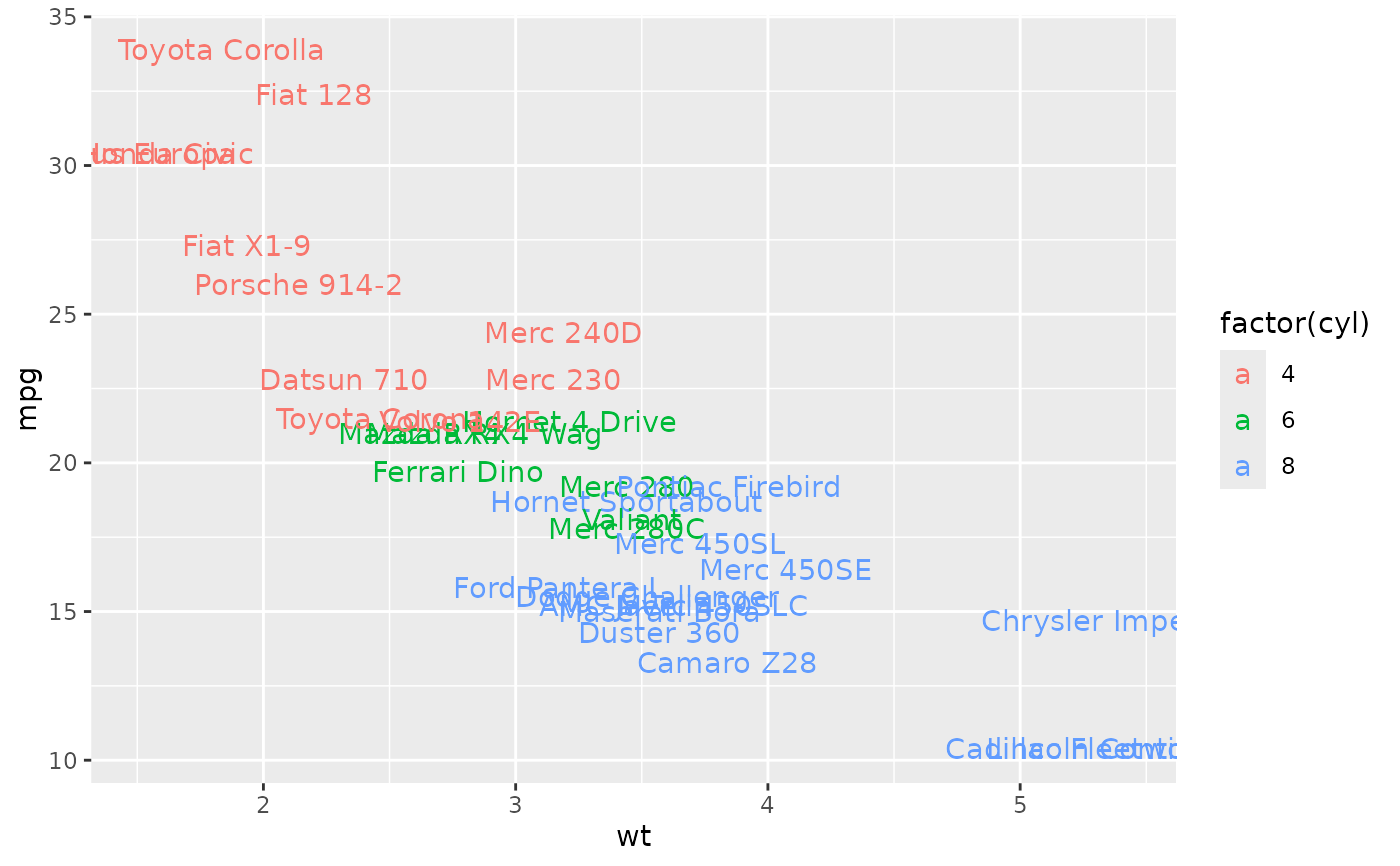p + geom_text(aes(colour = factor(cyl))) +
scale_colour_discrete(l = 40)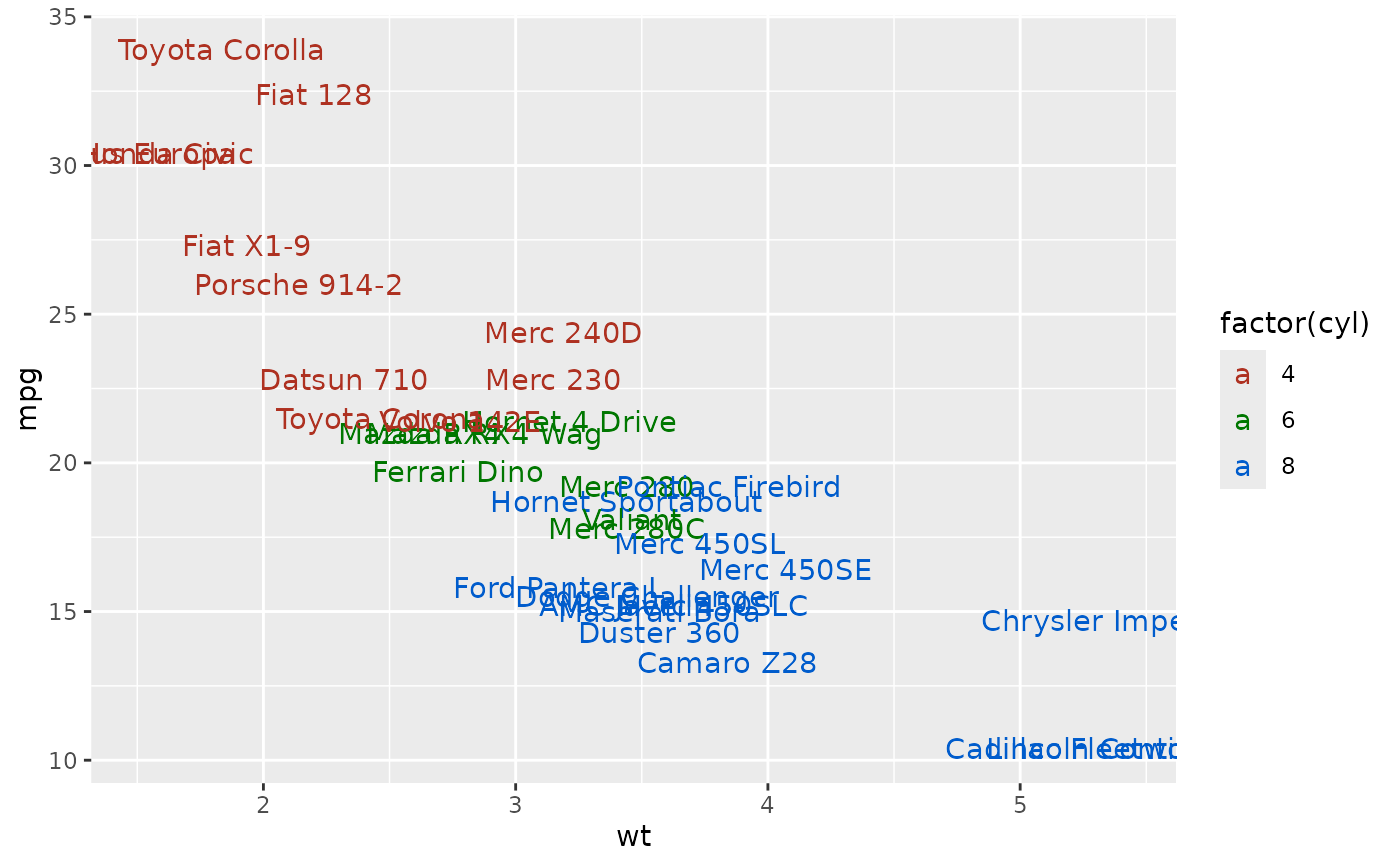p + geom_label(aes(fill = factor(cyl)), colour = "white", fontface = "bold")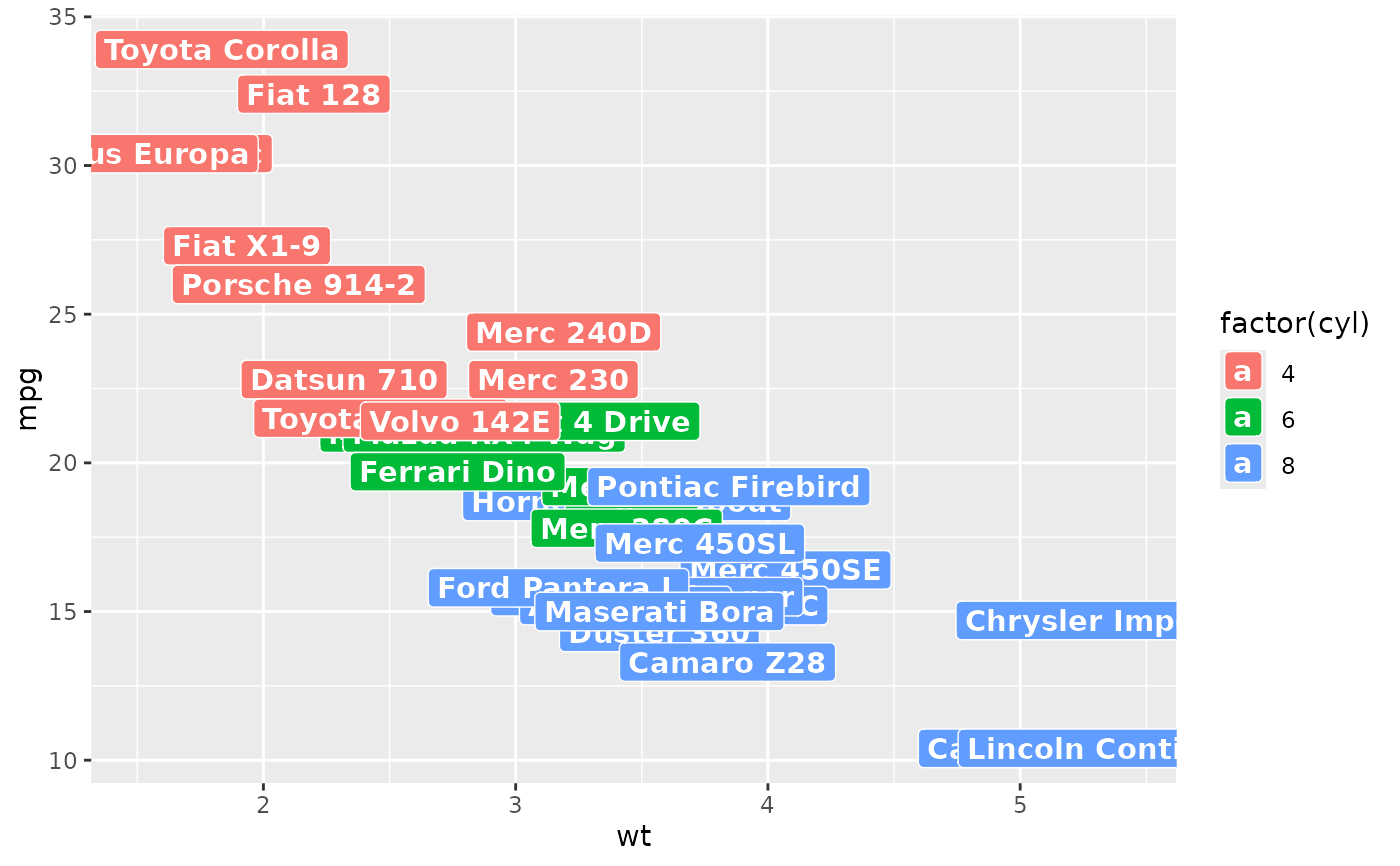p + geom_text(aes(size = wt))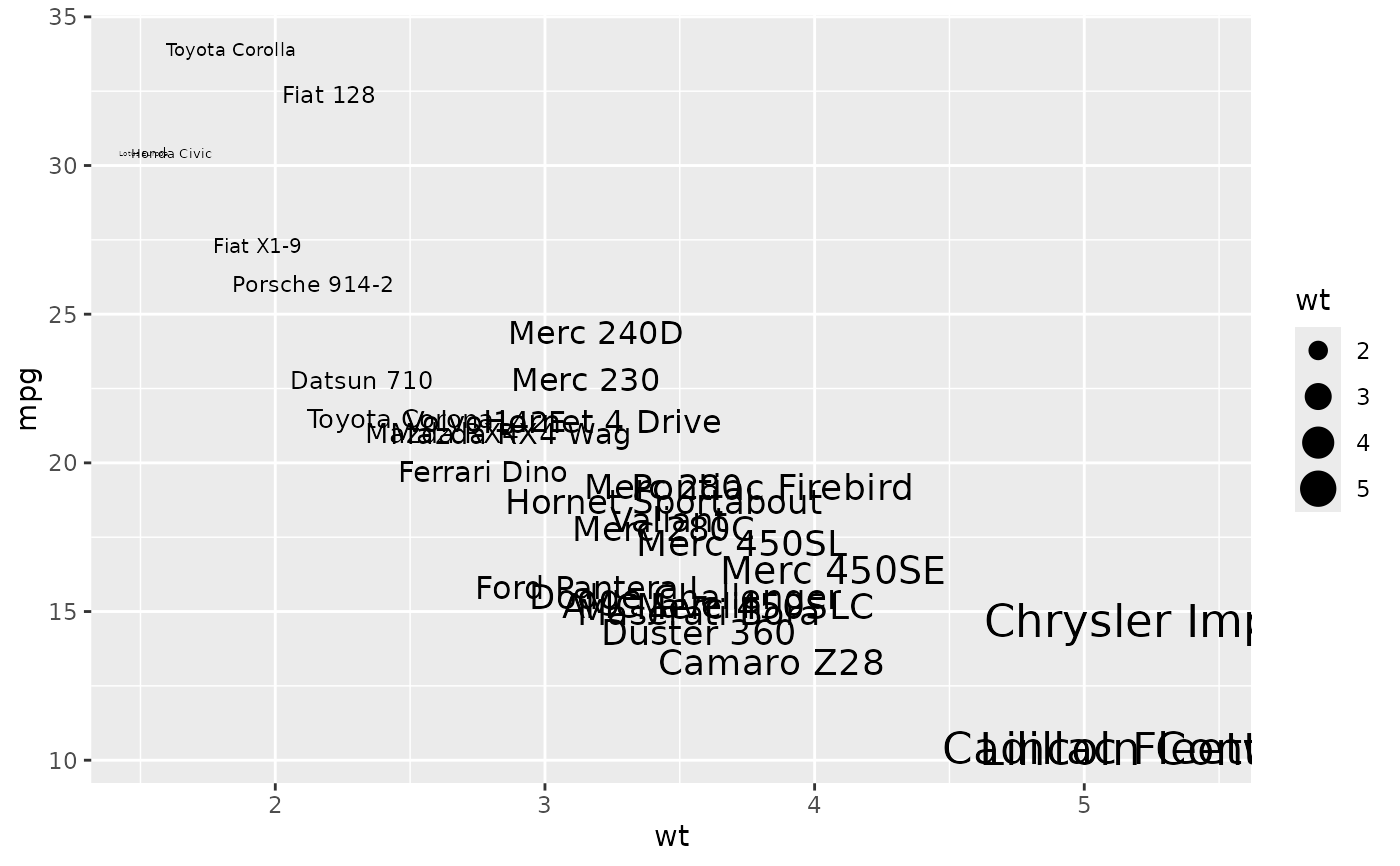# Scale height of text, rather than sqrt(height)
p +
geom_text(aes(size = wt)) +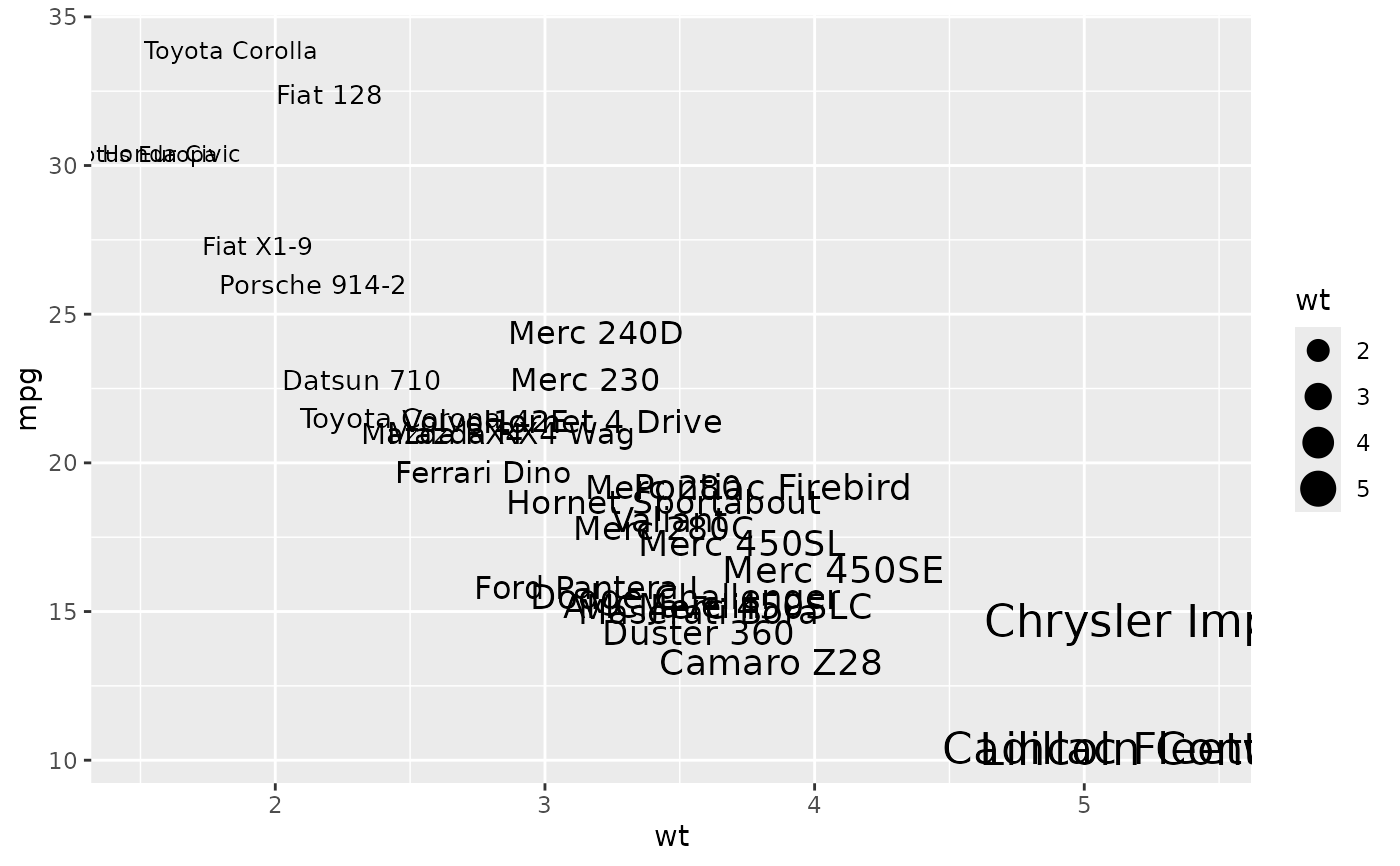# You can display expressions by setting parse = TRUE.  The
# details of the display are described in ?plotmath, but note that
# geom_text uses strings, not expressions.
p +
geom_text(
aes(label = paste(wt, "^(", cyl, ")", sep = "")),
parse = TRUE
)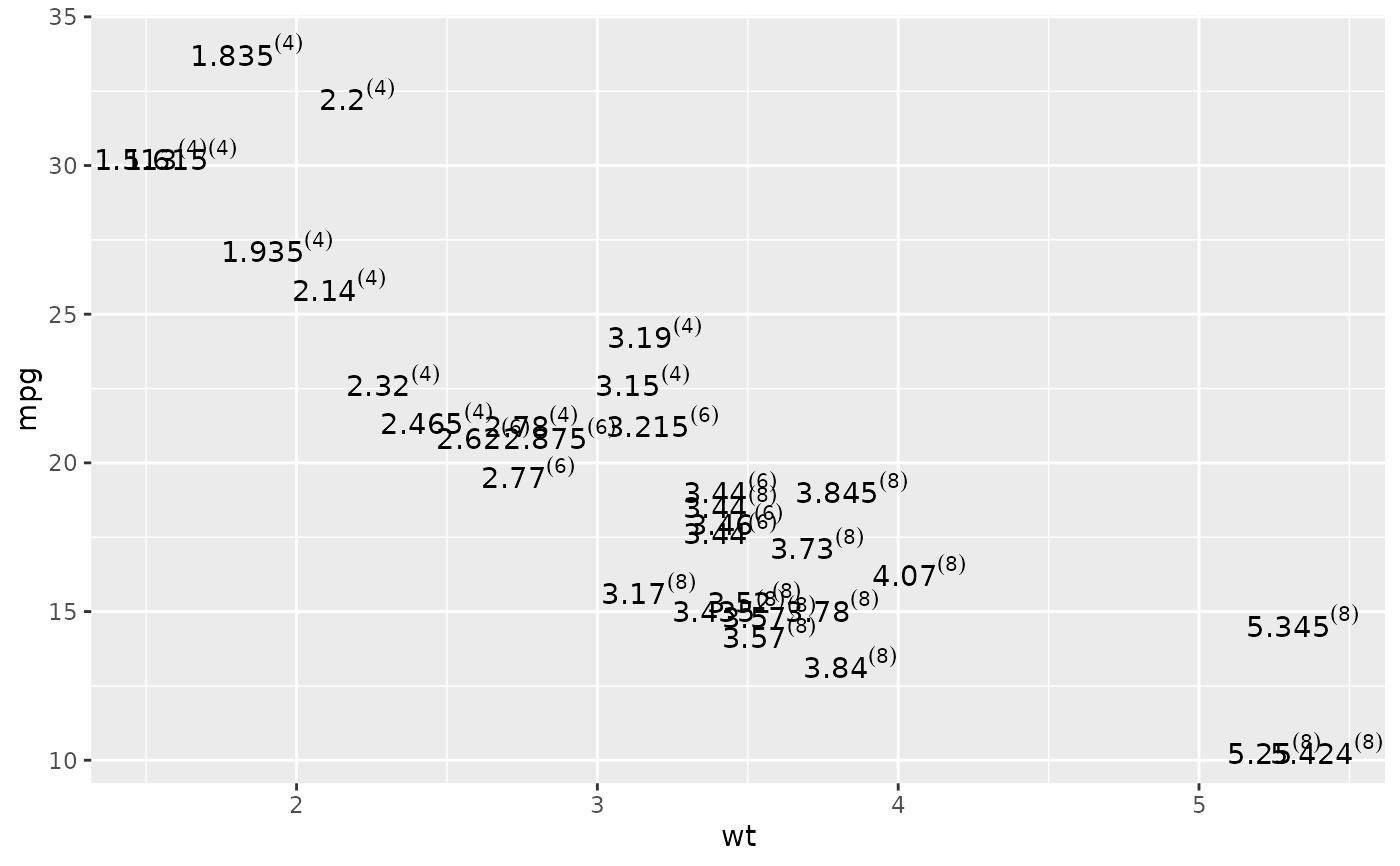p +
geom_text() +
annotate(
"text", label = "plot mpg vs. wt",
x = 2, y = 15, size = 8, colour = "red"
)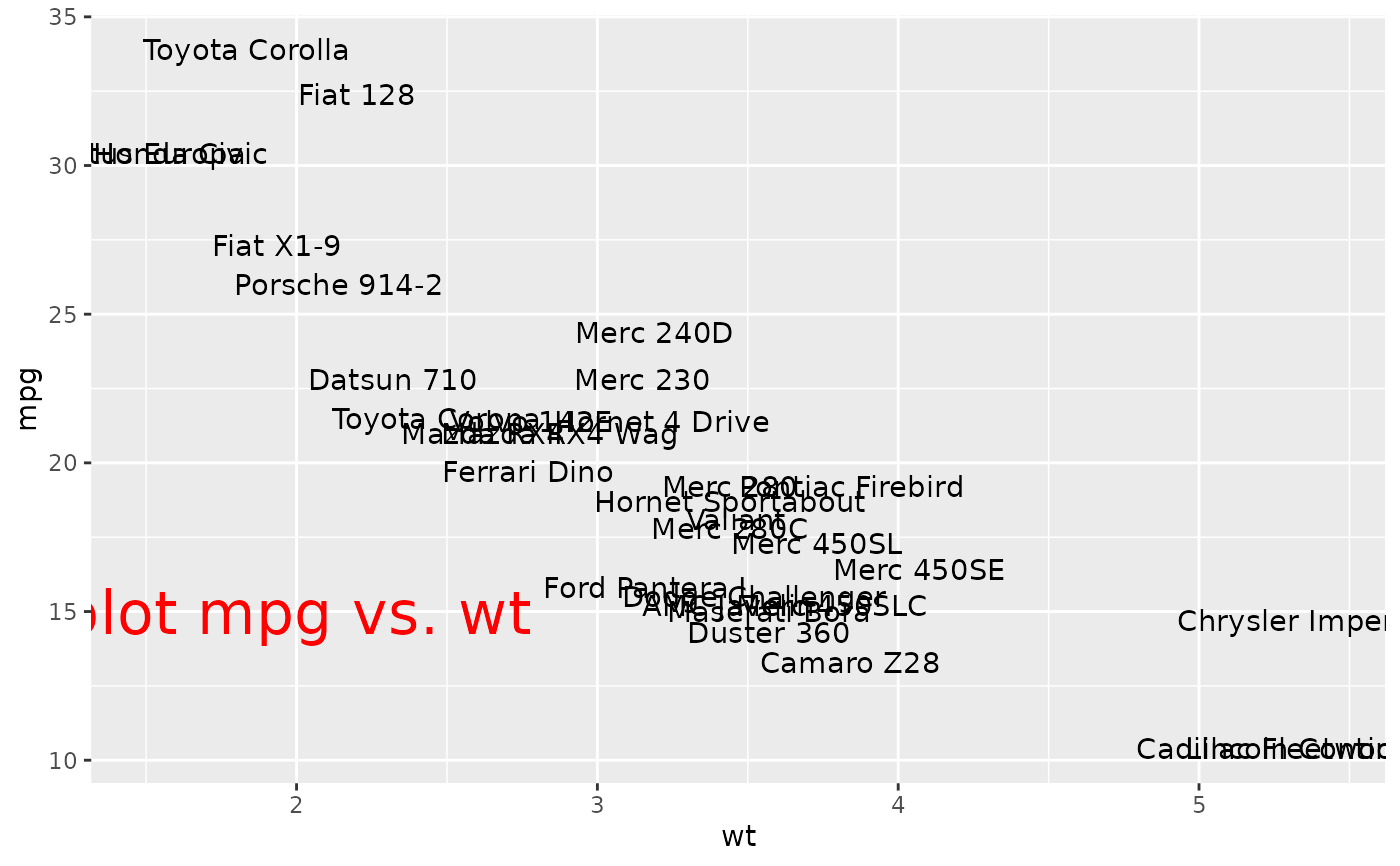# \donttest{
# Aligning labels and bars --------------------------------------------------
df <- data.frame(
x = factor(c(1, 1, 2, 2)),
y = c(1, 3, 2, 1),
grp = c("a", "b", "a", "b")
)

# ggplot2 doesn't know you want to give the labels the same virtual width
# as the bars:
ggplot(data = df, aes(x, y, group = grp)) +
geom_col(aes(fill = grp), position = "dodge") +
geom_text(aes(label = y), position = "dodge")
#> Warning: Width not defined. Set with position_dodge(width = ?)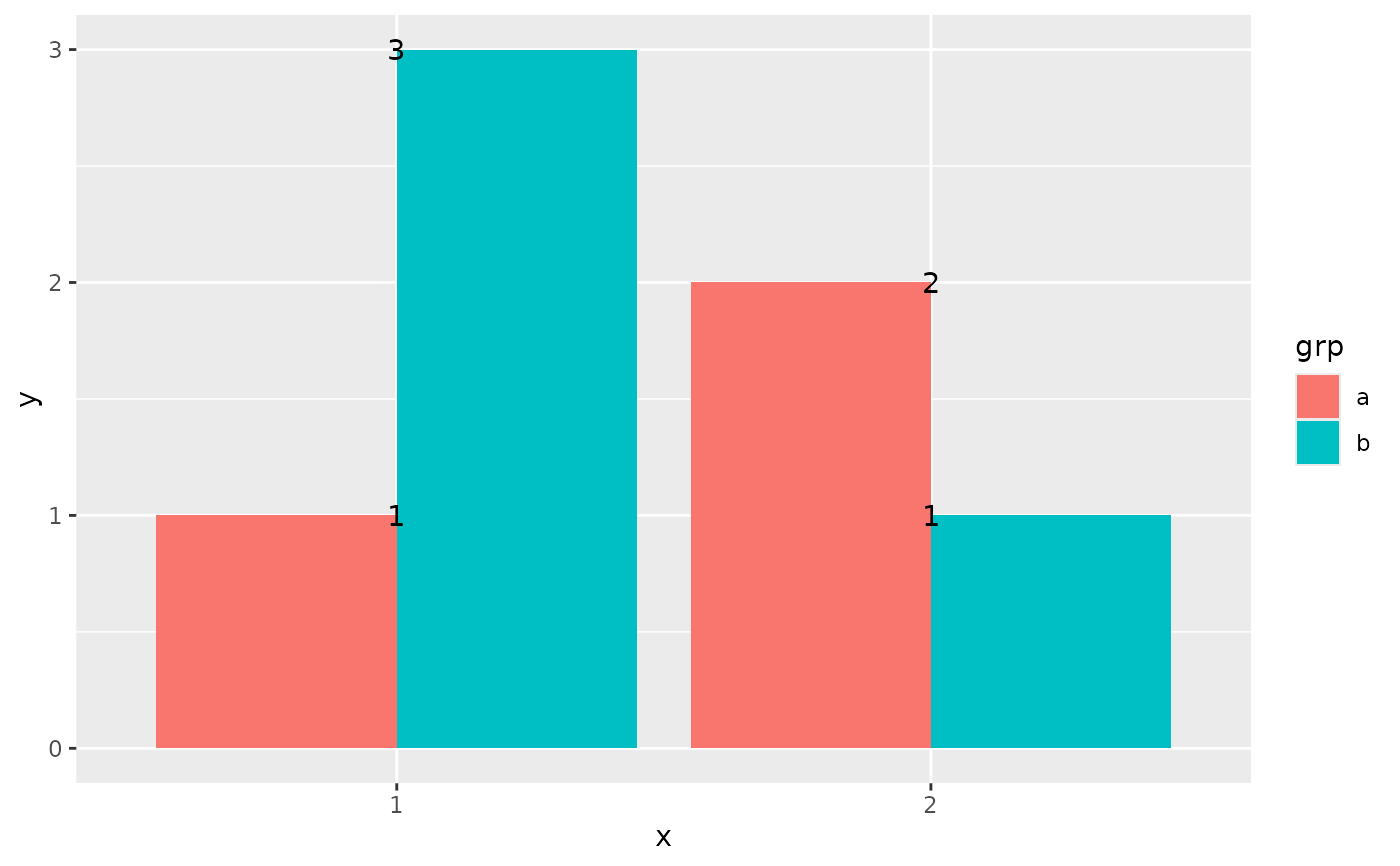# So tell it:
ggplot(data = df, aes(x, y, group = grp)) +
geom_col(aes(fill = grp), position = "dodge") +
geom_text(aes(label = y), position = position_dodge(0.9))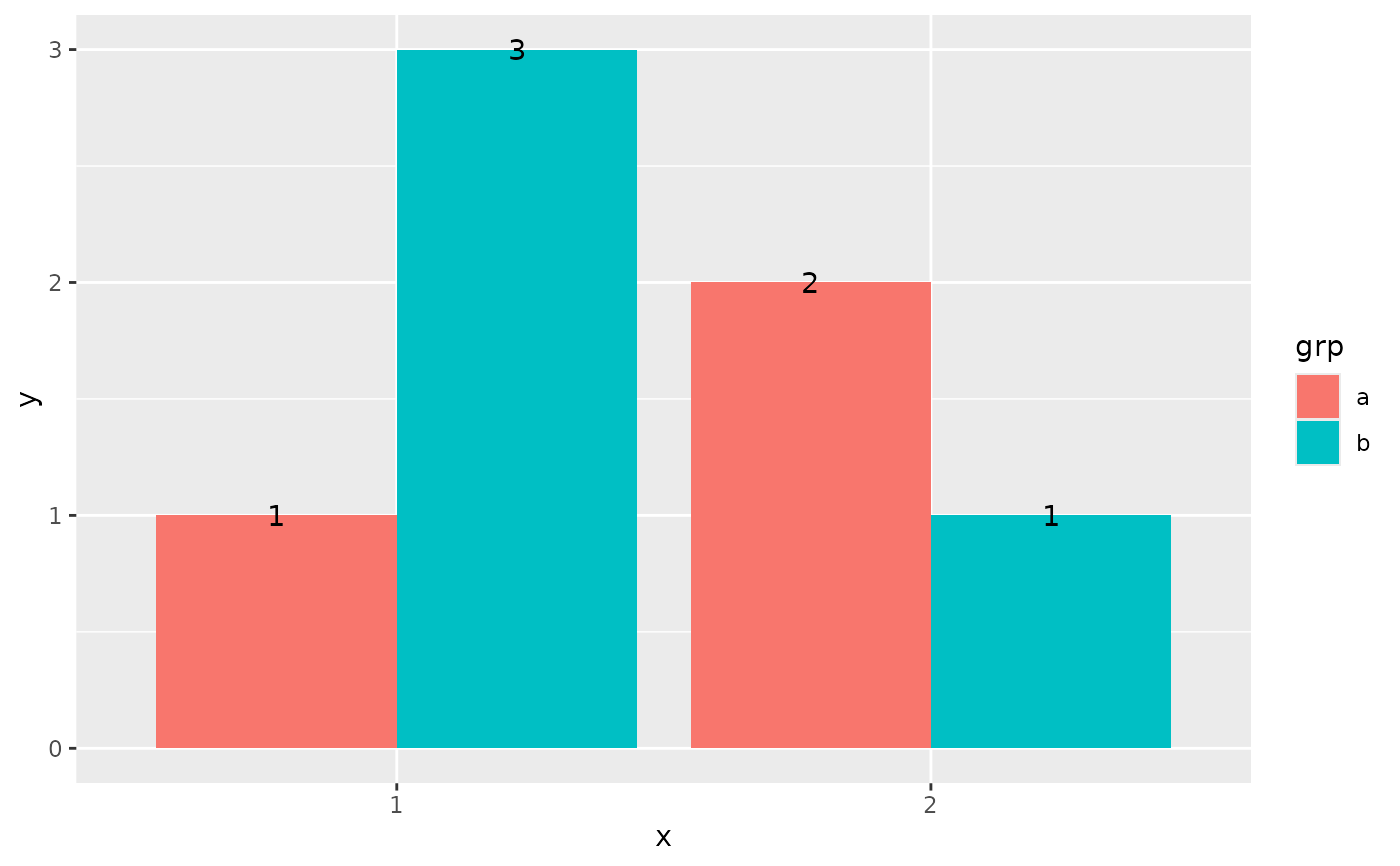# Use you can't nudge and dodge text, so instead adjust the y position
ggplot(data = df, aes(x, y, group = grp)) +
geom_col(aes(fill = grp), position = "dodge") +
geom_text(
aes(label = y, y = y + 0.05),
position = position_dodge(0.9),
vjust = 0
)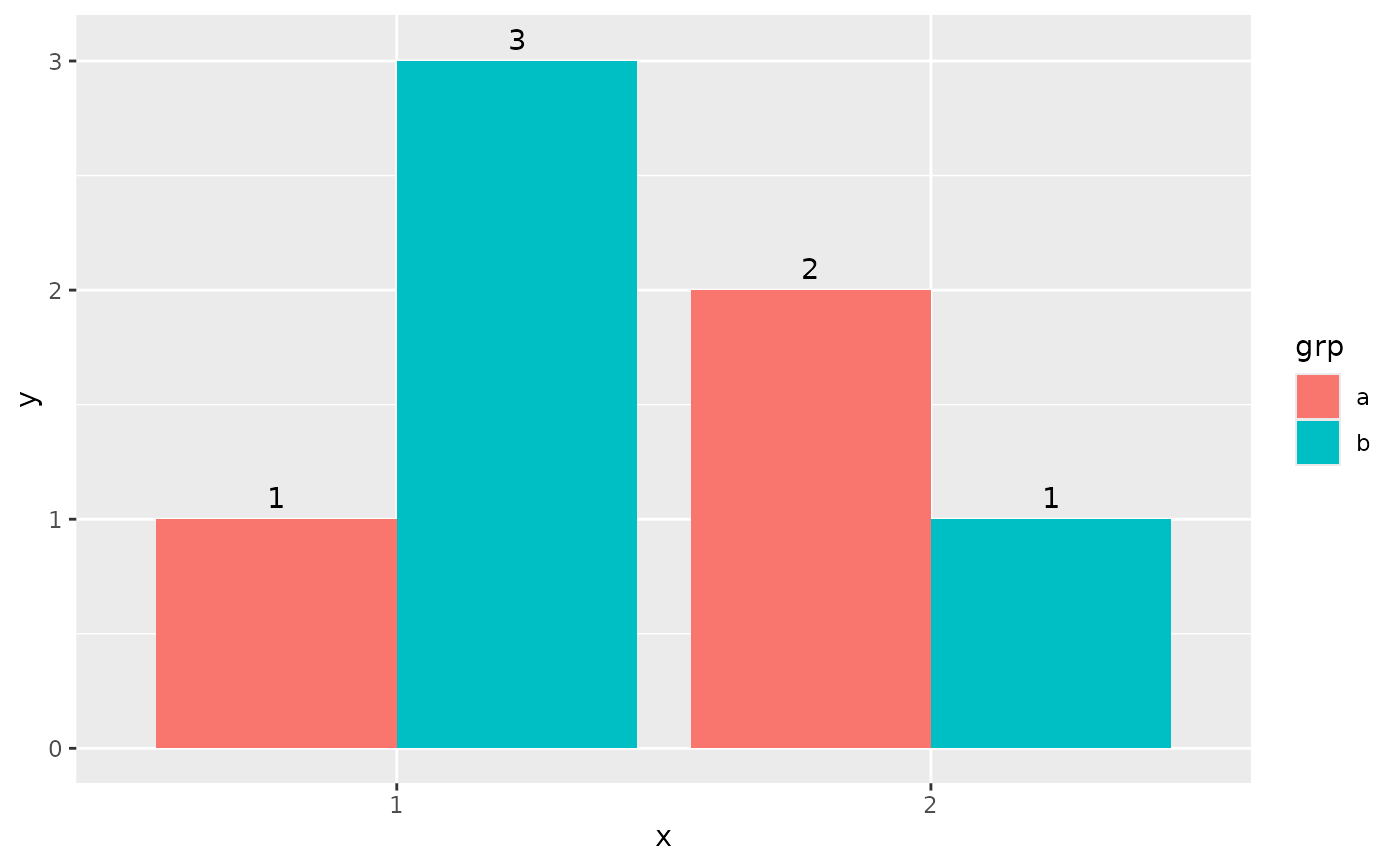# To place text in the middle of each bar in a stacked barplot, you
# need to set the vjust parameter of position_stack()
ggplot(data = df, aes(x, y, group = grp)) +
geom_col(aes(fill = grp)) +
geom_text(aes(label = y), position = position_stack(vjust = 0.5))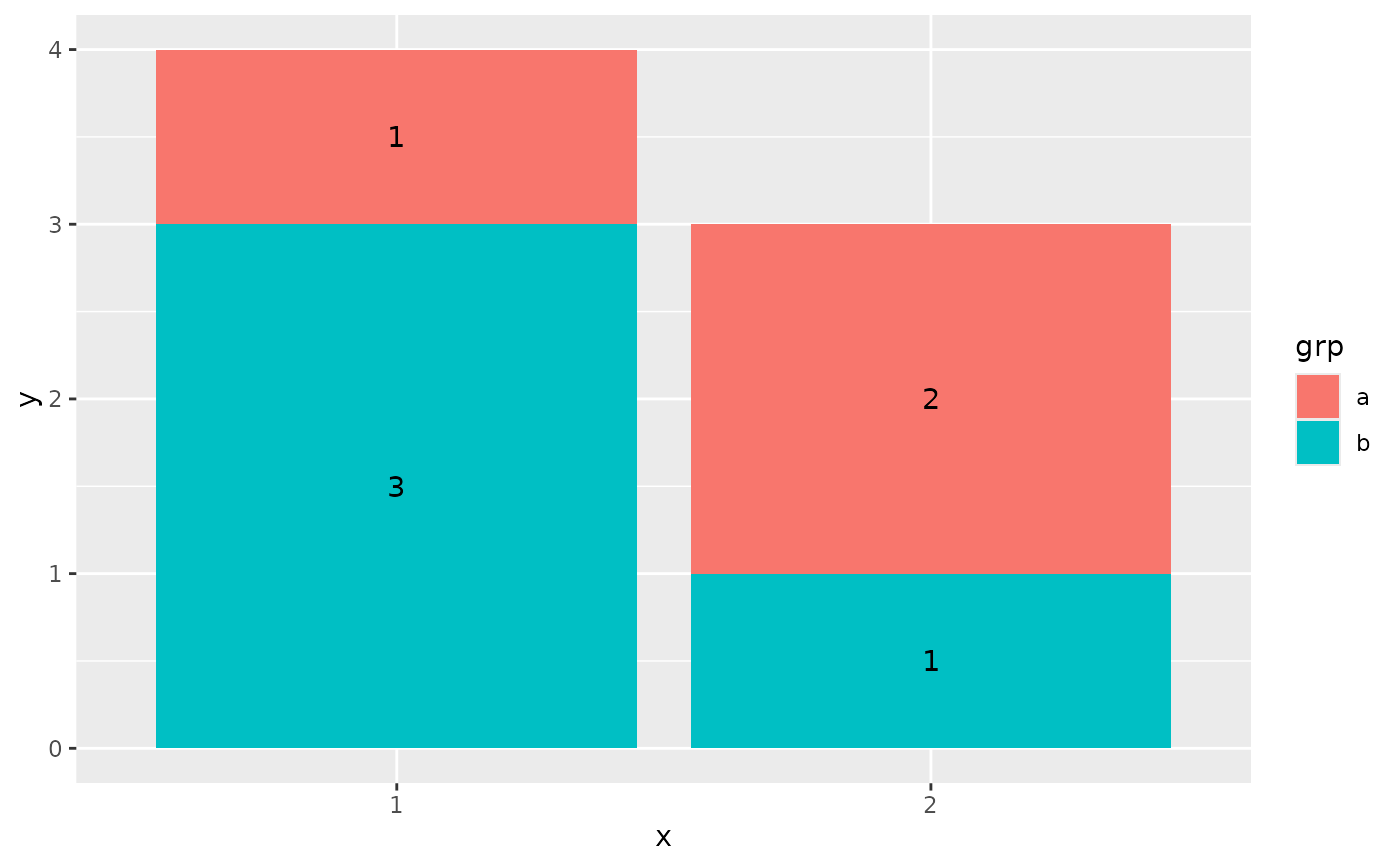# Justification -------------------------------------------------------------
df <- data.frame(
x = c(1, 1, 2, 2, 1.5),
y = c(1, 2, 1, 2, 1.5),
text = c("bottom-left", "bottom-right", "top-left", "top-right", "center")
)
ggplot(df, aes(x, y)) +
geom_text(aes(label = text))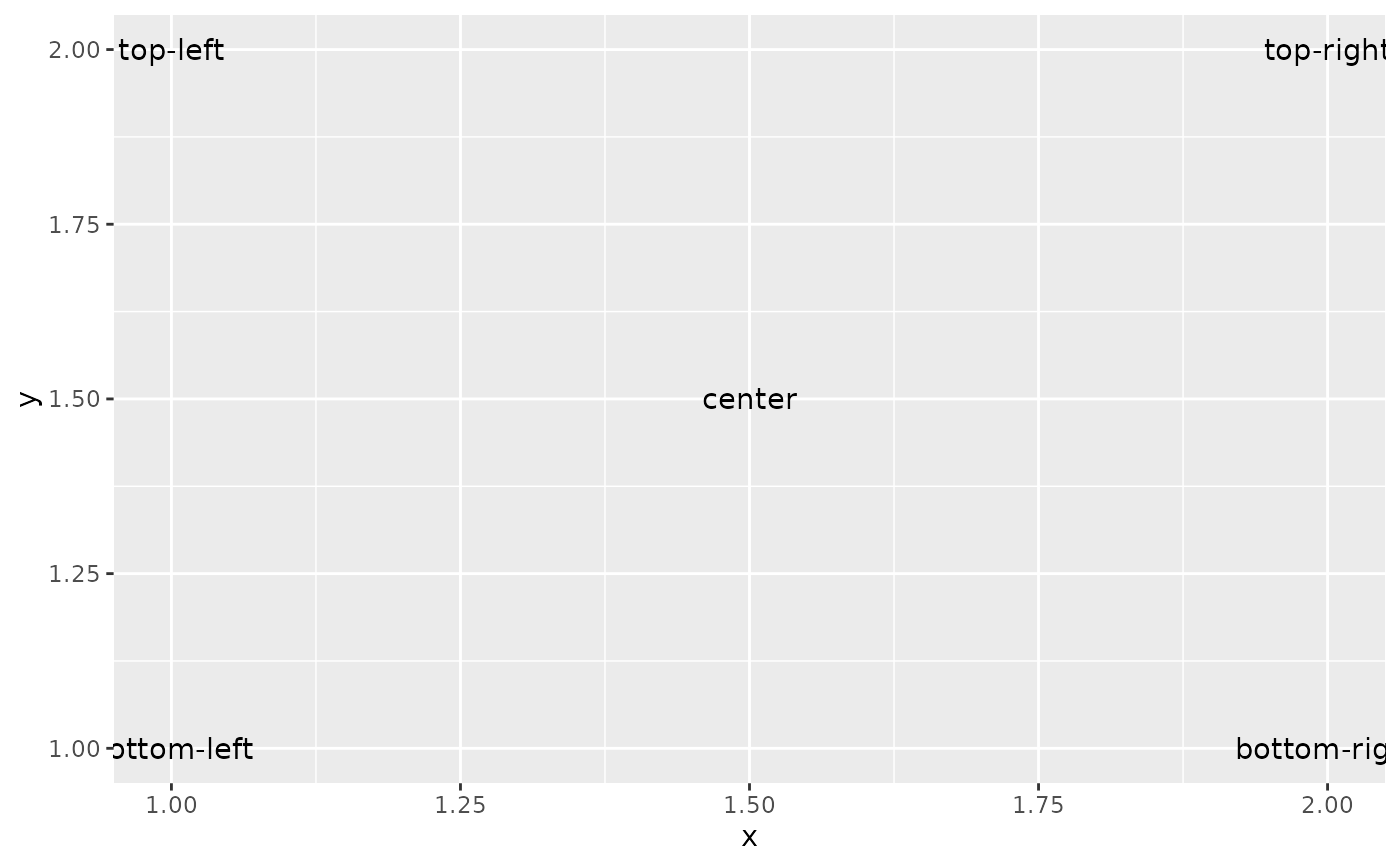ggplot(df, aes(x, y)) +
geom_text(aes(label = text), vjust = "inward", hjust = "inward")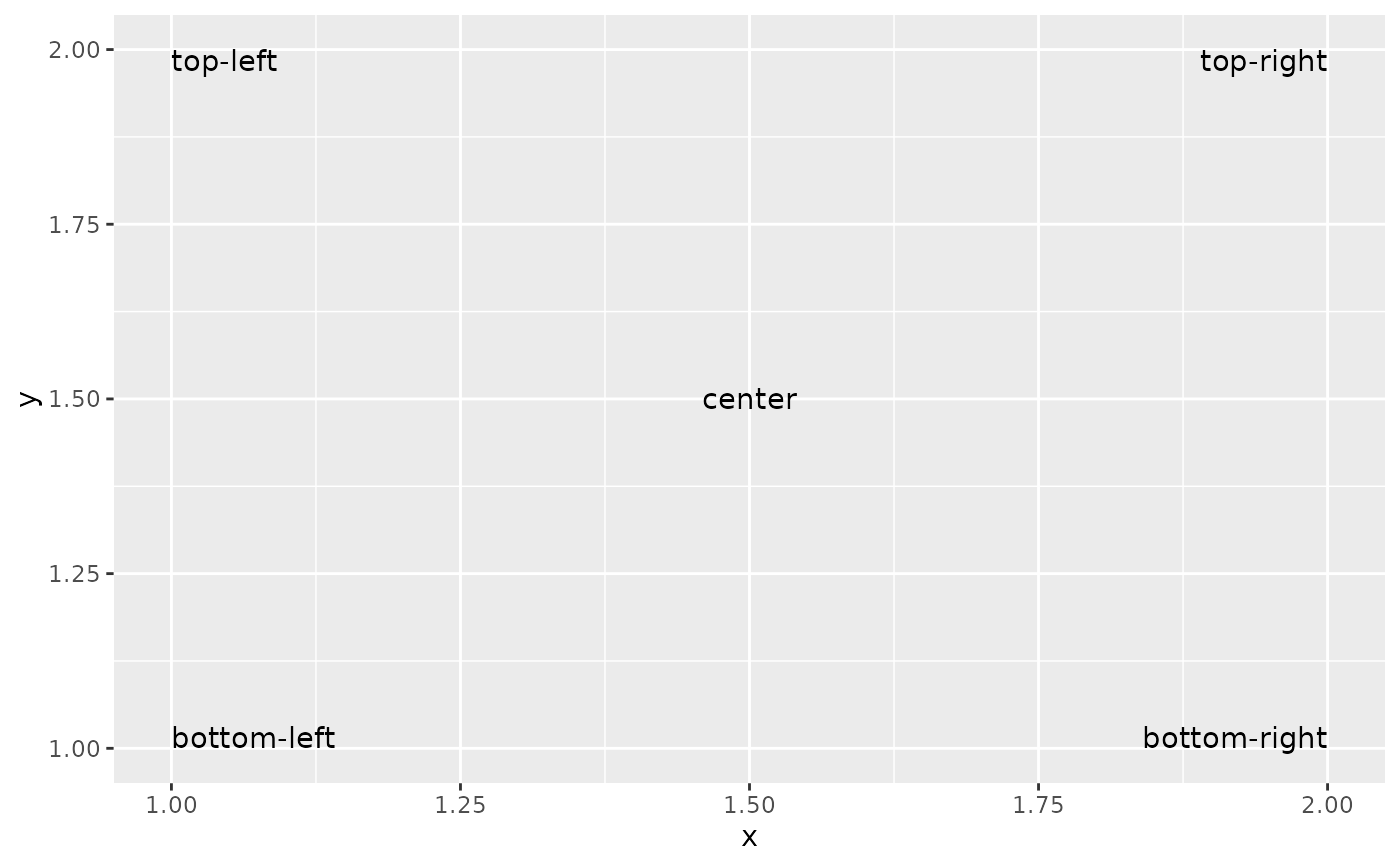# }